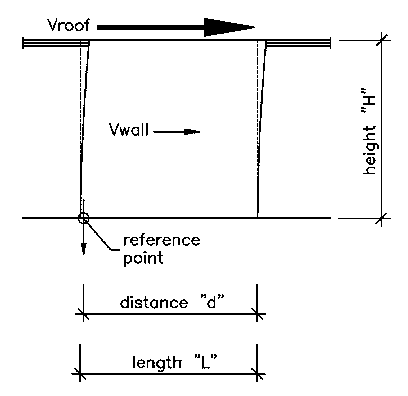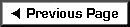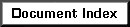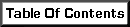A Wall To Resist Seismic LoadingWall Information: Vroof = 2600 pounds.  The weight of wall is 15 psf.  A roof contributes 150 plf to the top of this wall. The seismic coefficient is 0.183 and we are located in Zone 4.  Height = 12 feet. The wall is framed from 2x6 Douglas Fir No. 1 except as required. The Problem: We want to design a wall with a minimum width to resist the applied force.

• In Zone 4, wood shear walls are limited by a 2:1 ratio of height to width.  Therefore our minimum wall width is 6 feet.
• The total weight of the wall is (12)(6)(15 psf) = 1080 pounds.
• The seismic force in the wall (Vwall) is (1080)(0.183) = 197.64 pounds > Use 198 pounds.
• The overturning moment is (2600)(12) + (198)(6) = 32388 ft-lbs.
• The resisting moment absent hold-downs is the sum of the wall weight and the roof load times half the wall width or (1080 + (6)(150)) * (6/2) = 5940 ft-lbs.
• Since the overturning moment exceeds the resisting moment, a hold-down is required.
• The total unit shear in the wall is (2600 + 198) / 6 = 466.4 lbs./ft.  3-in. nominal framing will be required at panel edges.

We are going to need compression posts and hold-downs. One at a time...

Compression posts

• The end studs are going to be braced by sheathing for their entire length in the weak direction, therefore L/D = 144" / 5.5" = 26.18 (< 50, ok)
• Fc = (Cf for 6" stud width) * (Fc) = 1.1 * 1450 = 1595 psi
• Fce = (0.30* 1,700,000)/SQR(26.18) = 744.1 psi
• c = 0.80 for sawn lumber, so
• A = (1/1.6)*(1 + Fce/Fc) = 0.917
• B = (1/0.8)*(Fce/Fc) = 0.583
• Cp = A - SQRT( A*A - B) = 0.409
• Allowable unit load = (Cp) * (Fc) = (0.409) * (1595) = 652 psi (> Fc-perp. = 625 psi).
• Total allowable load not to exceed (1.5) * (5.5) * (625) = 5,156 pounds.

Hold-downs
Now calculate a rough hold-down (tiedown) force by subtracting the resisting moment from the overturning moment and dividing by an approximate distance to the hold-down (generally 6" from opposite end of wall).  Add this load to the vertical loads to calculate a rough compression...

• Approx. Tiedown = (32388 - 5940) / (5.5) = 4809 pounds.
• Approx. Compression = 4809 + 1080 + (6)(150) = 6789 pounds.
• The forces in this wall are caused by seismic loads, therefore we may increase the the allowable column loads by a factor of 1.33 (4/3).
• Checking a single 2 x 6... (5156) * (4/3) > 6789??... 6874 > 6789, ok.  As an engineer I would not allow such a small margin given the amount of force. Besides, we are forced to use a 3x stud at the edge of the shear wall due to the unit shear exceeding 350 pounds per foot.  A 3 x 6 would take (2.5)(5.5)(625)(4/3) = 11,458 pounds, ok.
• As for the hold-down, we will be bolting into a 3x edge member. Looking in the current Simpson Strong-Tie catalog, we find that an HD8A can resist 5,415 pounds in uplift.  This is our hold-down unless further calculations prove otherwise.
• The edge distance "d" in the diagram above is therefore ( L - (2.5" + 2.0625")) = 5.62 ft.

Now calculate the actual hold-down force...

• Mot = (Vroof * H) + (Vwall * H/2) = 32,388 ft-lbs.
• Mwall = (Weight of Wall + Weight of Roof)*(d-(L/2)) = (1080 + 900) * (5.62 - 3) = 5188 ft-lbs.
• The maximum compression per foot of wall is equal to the allowable load on the end post times (12/16) = 8593 pounds.  Later we will check adjacent studs as required to see if they are overstressed in compression.
• (Mot + Mwall) = (max. comp.)*(a)*(1.5)*(d-a) = 37,576 ft-lbs.
• "Constant" = (Mot + Mwall)/(max. comp. * 1.5) = (37,576)/(8593 * 1.5) = 2.915
• a = (d - SQRT((d*d) - (4 * Constant)))/2 = 0.578 feet
• Tiedown = (max. comp.)*(1.5 * a) - (Weight of Wall + Weight of Roof) = (8593)*(1.5)*(0.578) - 1980 = 5,470 lbs.
• Checking this force against the HD8A which is worth 5415 pounds we see that we need to goose the hold-down size up to an HD10A...its worth 6935 pounds.

The distance "a" (see previous diagrams) indicates the distance to the centroid of compression forces.  If "a" is large, we would check the stud adjacent to the compression post to see if it was overstressed in compression.  In this example, the distance (3*a) is 20.5 inches.  The first adjacent stud is very close to the end of the compression triangle. The total compression is 7450 pounds.  One third of that is 2483 pounds...well under our allowable for such a stud, ok.

Shear and Sheathing
Our unit shear in this wall is 466.4 lbs./ft.  As stated previously, the 1997 UBC will require 3x members at all panel edges. Since the wall is 12'-0" tall, we will also require 3x solid blocking at the 8'-0" elevation. In addition to the end posts, one 3 x 6 stud will be needed in the middle of the wall section.

The thickness of sheathing was not specified in the beginning, so we are left to select that as well as the nailing. Looking at Table 23-II-I-1 on page 2-288 of the 1997 UBC, we see our choices are:

• Structural I, 7/16" thick, with 8d nails at 3" o.c. at panel edges, 12" o.c. in the field, for 505 plf.
• Structural I, 15/32" thick, with 10d nails at 4" o.c. at panel edges, 12" o.c. in the field, for 510 plf.
• C-D, C-C Sheathing, 15/32" thick, with 8d's at 3" o.c. at panel edges, 12" o.c. in the field, for 490 plf.
• C-D, C-C Sheathing, 15/32" thick, with 10d's at 3" o.c. at panel edges, 12" o.c. in the field, for 600 plf.
• C-D, C-C Sheathing, 19/32" thick, with 10d's at 4" o.c. at panel edges, 12" o.c. in the field, for 510 plf.

Sill Bolting
If the bottom plate of this shear wall sits directly on top of a foundation stem wall, there is an additional step: bolting the sill down for lateral loads. We want to use bolts that will have at least four diameters of edge distance on the bottom plate so these same bolts can resist perpendicular forces. This means we should use a bolt diameter less than (5.5" / 8) or 5/8".  The Code generally calls for 1/2" bolts so that is what we will use.

Looking at Table 23-III-B-1 on page 2-296, we see that 1/2" diameter bolts do not have a listed value in 2 1/2" thick material.  The value in 1 1/2" material, parallel to grain, is 480 pounds.  The value for 3 1/2" material is 610.  The Code allows interpolation in these instances, therefore the allowable shear is (480 + 610)*(0.5) = 545 pounds.  Because the forces are the result of earthquake loads, we can increase this value by a factor of 4/3 = 726 pounds per bolt.

6 feet * 466.4 = 2798.4 lbs.

(2798.4) / (726) = 3.85 bolts > Use four 1/2" diameter bolts.  Space them at 16" centers near the interior of the wall to leave room for the hold-down bolts at the ends.